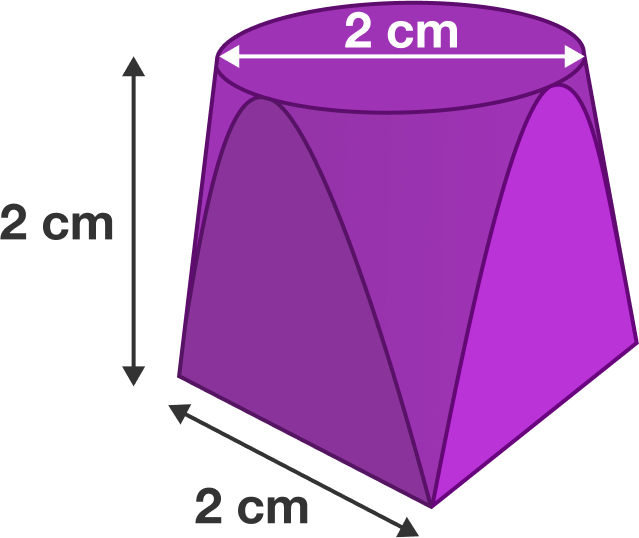# Lindsay's shape

Calculus Level 3

After studying various 3D shapes and finding formulas for their volumes, I challenged my students to invent a new shape. Lindsay created a shape $($with height $2 \text{ cm})$ that is circular at the top $($with radius $1\text{ cm})$ but square at the bottom $($with side length $2\text{ cm}).$ Lindsay created this shape from a paraboloid: sliced four times parallel to the paraboloid's axis and two times perpendicular to the paraboloid's axis. Lindsay's shape is pictured below.

Find the volume of Lindsay's shape in $\text{cm}^{3},$ which can be written as $\frac{A}{B}+C\pi$ with $A,B,C$ integers, $A$ and $B$ coprime, and $B$ positive.

Give the value of $A+B+C.$×

Problem Loading...

Note Loading...

Set Loading...